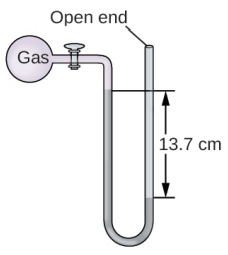# Problem: The pressure of a sample of gas is measured at sea level with an open-end mercury manometer. Assuming atmospheric pressure is 760.0 mm Hg, determine the pressure of the gas in:(c) kPa

⚠️Our tutors found the solution shown to be helpful for the problem you're searching for. We don't have the exact solution yet.

###### Problem Details

The pressure of a sample of gas is measured at sea level with an open-end mercury manometer. Assuming atmospheric pressure is 760.0 mm Hg, determine the pressure of the gas in:

(c) kPa